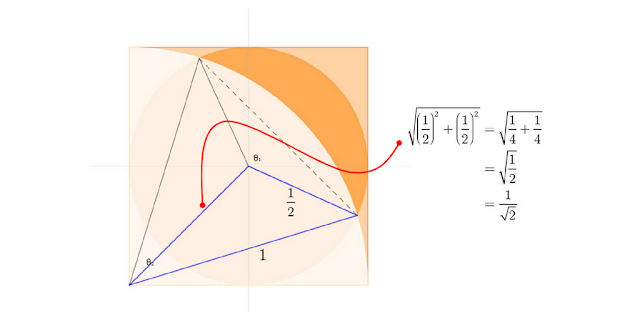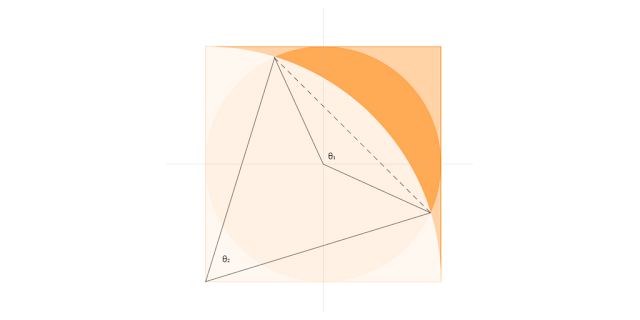## Friday, 24 November 2017

### On the Crescent Area ProblemProblem... A circle is inscribed in a square that has a side length of one unit, which is also the radius of a quarter-circle.  Find the area of as many of the different shapes and regions that you can see.  (Which shapes, or regions, are you finding difficult?  What is it that is stopping you find their areas?)

Students typically define the square, the inscribed circle, the quarter circle and often a quarter of the inscribed circle as shapes and regions to find the areas of, and proceed to do so without issue.  Students also note the dark orange 'crescent' region as an area to find, and, indeed, the lighter orange regions around it, and these delineate the aspects of the problem that have the potential to develop into the most formative discussions.

Students, with the teacher's guidance / encouragement of course, can outline a strategy of how to find these more complex areas, ignoring whether or not they yet have the knowledge, skills or understanding to follow such a strategy through.  Depending on where students are in their mathematical careers, this can then lead into more direct teaching, or, moreover, can function as a segue into a body of work that will provide students with the knowledge and skills they need to apply their strategy.

To see a 'thinking through' of a typical solution / approach with students to the problem of the 'crescent' area, click on 'Read more' below.

Suggested Solution:

To find the Area of the dark orange ‘crescent’ shape (A), start by defining Aas the area for the segment defined by θ1 and A2 as the area for the segment defined by θ2.  (The opening up of one way to a solution hinges on this idea for most students.  It is worth, therefore, deliberating upon in some depth with students in terms of how we can see that the crescent area is the difference in the areas of the respective segments.)  Then, using students' knowledge of finding the area of a segment of a circle:

\begin{array}{c}\begin{align}A &= {A_1} - {A_2}\\\\{A_1} &= \frac{{{\theta _1}}}{{360}}\pi {\left( {\frac{1}{2}} \right)^2} - \frac{1}{2}{\left( {\frac{1}{2}} \right)^2}\sin {\theta _1}\\{A_1} &= \frac{{\pi {\theta _1}}}{{1440}} - \frac{{\sin {\theta _1}}}{8}\\\\{A_2} &= \frac{{\pi {\theta _2}}}{{360}} - \frac{{\sin {\theta _2}}}{2}\end{align}\end{array}

To find θ1 we can use the cosine rule, but of course, whilst we know the radius of the inscribed circle to give us the two equal sides in the isosceles triangle containing θ1, we don't know the length of the side opposite it; of the chord of the inscribed circle.  However, we know (or can easily show) that given an angle, say p, $$\begin{array}{c}\cos p = - \cos \left( {180 - p} \right)\end{array}$$, e.g. $$\cos 38 = 0.788...\;\therefore \cos 142 = - 0.788...$$, so we can use this to help us.  (This is likely to be another crucial point to the solution for many students who are at a position to be able to attempt it.  It is a key aspect to moving forward in learning for them and should be considered with them thoughtfully, deliberately.)

So considering the triangle defined by the angle (180 – θ1/2), we can find θ1:

\begin{array}{c}\begin{align}\cos \left( {\frac{{{\theta _1}}}{2}} \right) &= - \cos \left( {180 - \frac{{{\theta _1}}}{2}} \right)\\\\\cos \left( {180 - \frac{{{\theta _1}}}{2}} \right) &= \frac{{{{\left( {\frac{1}{2}} \right)}^2} + {{\left( {\frac{1}{{\sqrt 2 }}} \right)}^2} - 1}}{{2 \times \frac{1}{2} \times \frac{1}{{\sqrt 2 }}}}\\ &= \frac{{ - \frac{1}{4}}}{{\frac{1}{{\sqrt 2 }}}}\\ &= - \frac{{\sqrt 2 }}{4}\\\cos \left( {\frac{{{\theta _1}}}{2}} \right) &= - \left( { - \frac{{\sqrt 2 }}{4}} \right)\\\frac{{{\theta _1}}}{2} &= 69.29518894536...\end{align}\end{array}

So we now have θ138.5903778907...° where — to emphasise — the lengths in the triangle we used were the radius of the inscribed circle, the radius of the quarter circle, and the length of the diagonal in the bottom left square (i.e. from Pythagoras):At this point, 'forgetting' their strategy, students may typically notice that by using trigonometry, we are able to find the chord length thus:

\begin{array}{c}\begin{align}\sin \left( {\frac{{{\theta _1}}}{2}} \right) &= \frac{{\frac{1}{2}{\rm{ chord}}}}{{\frac{1}{2}}}\\\frac{1}{2} \times \sin 69.295... &= \frac{1}{2}{\rm{ chord}}\\\\{\rm{∴ chord}} &= 0.935414346693...\end{align}\end{array}

Students may then reason that this is not particularly helpful, and look back at where they are in the solution, namely that we now have θand thus will (or should) be able to find the areas of the respective segments Aand A2:

\begin{array}{c}\begin{align}{A_1} &= \frac{{\pi {\theta _1}}}{{1440}} - \frac{{\sin {\theta _1}}}{8}\\ &= \frac{{\pi \times 138.59...}}{{1440}} - \frac{{\sin 138.59....}}{8}\\ &= 0.302... - 0.082...\\ &= 0.219676572251...\end{align}\end{array} and
${A_2} = \frac{{\pi {\theta _2}}}{{360}} - \frac{{\sin {\theta _2}}}{2}$but of course we need to find θ2......which is when students realise that the chord length they worked out earlier is indeed a part of their solution, thus:

\begin{array}{c}\begin{align}\cos {\theta _2} &= \frac{{1 + 1 - {{\left( {0.935414346693...} \right)}^2}}}{{2 \times 1 \times 1}}\\\cos {\theta _2} &= 0.5625\\{\theta _2} &= 55.77113367216...\end{align}\end{array}

Hence, having found A1 and having everything  we require to find A2: \begin{array}{c}\begin{align}{A_2} &= \frac{{\pi \times 55.77113367216...}}{{360}} - \frac{{\sin 55.77113367216...}}{2}\\ &= 0.486694955074... - 0.413398642354...\\ &= 0.07329631272...\end{align}\end{array}

And thus the area A of the dark orange 'crescent' region:
\begin{array}{c}\begin{align}A &= 0.219676572251... - 0.07329631272...\\ &= 0.146380259531...\end{align}\end{array}

(Once this crescent area is found, areas of other regions in the diagram are easily found.)

Of course, to emphasise, this approach is one that can be accessed by students studying to GCSE, and is a good example of a problem that can support the transition to more advanced mathematics in KS5 — in the sense of students being encouraged to evaluate the solution from an aesthetic viewpoint.  In other words, students could consider what aspects of the solution seem to be the most efficient, 'clever', etc., and what aspects come across as 'ugly', inefficient, problematic.  The recording of so many intermediary steps, for example, and the necessary use of numbers in decimal form — can we work out a way of solving the problem that removes the need to record results in such a form and thus assures a higher degree of exactitude in the final result?  Such questioning would thus leading into work to develop the generalisation that for any circular arc with radius r, where the half-angle subtended by the arc is θ, the area of corresponding circular segment is given by:

\begin{array}{c}\begin{align}A &= {r^2}\theta \left( {\sin \theta \cos \theta } \right)\\ &= {r^2}{\sin ^{ - 1}}\frac{\ell }{r} - \ell \left( {{r^2} - {\ell ^2}} \right)\\ &= {r^2}{\sin ^{ - 1}}\frac{{r\sin \theta }}{r} - r\sin \theta \left( {{r^2} - {{\left( {r\sin \theta } \right)}^2}} \right)\end{align}\end{array}